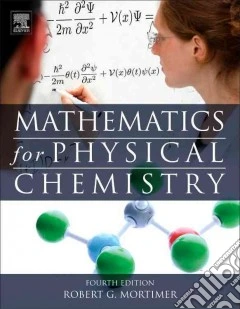ricerca
avanzata

# Mathematics for Physical Chemistry

## Un libro in lingua di Mortimer Robert G. edito da Elsevier Science Ltd, 2013

• € 49,70
• Il prezzo è variabile in funzione del cambio della valuta d’origine"Principal Facts and Ideas. Problem solving is the principal tool for learning physical chemistry. Problem solving can be approached in a systematic way. Many problems involve numerical calculations involving measurable quantities. A measured quantity consists of a number and a unit of measurement. The SI units have been officially adopted by international organizations of physicists and chemists. Consistent units must be used in any calculation. The factor-label method can be used to convert from one unit of measurement to another. Reported values of all quantities should be rounded so that insignifi- cant digits are not reported. Objectives After you have studied the chapter, you should be able to: analyze a problem and design a procedure for solving the problem; 4 1. Problem Solving and Numerical Mathematics carry out the numerical procedures use in solving a simple problem; use numbers and units correctly to express measured quantities; understand the relationship of uncertainties in measurements to the use of significant digits; use consistent units, especially the SI units, in equations and formulas; use the factor-label method to convert from one unit of measurement to another. 1.1 Problem Solving Techniques of problem solving are applicable to many intellectual areas. There is a useful little book on problem solving by G. Polya, 1 and much of our discussion of problem solving is based on this book. Most physical chemistry problems are stated verbally, like the so-called 'word problems' of elementary school. The information contained in the statement of the problem generally includes a statement of the physical system involved, some information about the state of the system, and a statement of the desired outcome"--